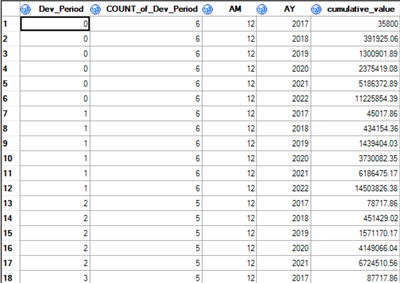## Identify variable value for succeeding combination of variablesIn ascending order for all sorting variables (Dev_Period, AM, AY).

I want to create a loop.

For example, when Dev_Period = 1, Count_of_Dev_Period = 6, then I want to sum the first 6 values of cumulative_value when Dev_Period = 0, then use that sum as the denominator. I want to sum all values of cumulative_value when Dev_Period = 1 as the numerator.

For example, when Dev_Period = 2, Count_of_Dev_Period = 5, then I want to sum the first 5 values of cumulative_value when Dev_Period = 1, then use that sum as the denominator. I want to sum all values of cumulative_value when Dev_Period = 1 as the numerator.

So on and so forth.

I haven't produced any code yet, but the code ChatGPT generates is beyond useable, so if anyone has any ideas, please advise. Thank you so much!

2 REPLIES 2

## Re: Identify variable value for succeeding combination of variables

I'm not surprised that Chatgpt generates unusable code. I do not see an actual clear description of the rules involved. 2 Examples are not a generic rule.

For example when you say " when Dev_Period = 1, Count_of_Dev_Period = 6," what is in the data that says you want to use values of Cumulative_value when Dev_period=0?

Similar for " when Dev_Period = 2, Count_of_Dev_Period = 5" what in  the data says to use the values of Cumulative_value when Dev_period is 1?

I am asking about the general rule because I am not sure what to do with Dev_period=3. Is it supposed to use Dev_period1 values? Dev_period2 values? Dev_period 1 and 2 both? And asking about what in the data determines which values to use because I can program something will do exactly what you say for the exact given description. But it only will do you example because a general program needs to know where to look for ALL related values and how to use them. This might have been where Chatgpt gagged on instructions.

If this is a general problem do you have a description or name associated? Sometime folks try to implement pieces of a larger problem one bit at a time and, especially new SAS users, don't realize that some other procedure implements the whole problem and you don't need to do this manually.

Instructions here: https://communities.sas.com/t5/SAS-Communities-Library/How-to-create-a-data-step-version-of-your-dat... will show how to turn an existing SAS data set into data step code that can be pasted into a forum code box using the </> icon or attached as text to show exactly what you have and that we can test code against.

PS: Can't program from pictures. Not even going to attempt to create such a data set by retyping. Provide data examples as a data step. If a variable isn't needed for the program, don't bother to include it. You don't shown any need for AM and AY so don't bother with them, OR describe how they are important because some solutions to the given calculation may obscure them.

Also, if you created this data by summarizing something it may be that the raw more granular data is actually useful. See "is there a name associated with this process" above.

@yp2609 wrote:In ascending order for all sorting variables (Dev_Period, AM, AY).

I want to create a loop.

For example, when Dev_Period = 1, Count_of_Dev_Period = 6, then I want to sum the first 6 values of cumulative_value when Dev_Period = 0, then use that sum as the denominator. I want to sum all values of cumulative_value when Dev_Period = 1 as the numerator.

For example, when Dev_Period = 2, Count_of_Dev_Period = 5, then I want to sum the first 5 values of cumulative_value when Dev_Period = 1, then use that sum as the denominator. I want to sum all values of cumulative_value when Dev_Period = 1 as the numerator.

So on and so forth.

I haven't produced any code yet, but the code ChatGPT generates is beyond useable, so if anyone has any ideas, please advise. Thank you so much!

## Re: Identify variable value for succeeding combination of variables

I would be curious to understand what you are trying to calculate.

The sum of a cumulative sum sounds like a very strange statistic to create.

Discussion stats
• 2 replies
• 115 views
• 0 likes
• 3 in conversation